﻿ 多馈入高压直流换向失败与交流传输线导纳关系的研究

# 多馈入高压直流换向失败与交流传输线导纳关系的研究Study on the Relationship between the Commutation Failure of Multi-InfeedHVDC Transmission and AC Transmission Line Admittance

Abstract: In this paper, two AC/DC parallel multi infeed HVDC transmission system models are established. Based on the basic knowledge of circuit theory, the expressions of admittance and commutation voltage of AC transmission line with multi infeed and DC parallel connection are derived. In addi-tion, the relationship between the admittance and the commutation failure of the AC transmission line is studied in detail. From the theoretical analysis that the inverter commutation failure admittance and transmission line relations are very complex, it is not only the equivalent admittance of AC power supply and DC transmission electronic system connected to the inverter side AC power supply, but also with the electronic rectifier DC transmission system connected to the value of admittance.

1. 引言

2. 数学模型的建立及机理分析

2.1. 典型系统结构模型

2.2. 直流输电系统逆变侧对应的交流系统母线电压的计算

$\left({E}_{1}-{U}_{ac1}\right){y}_{N1}=\left({U}_{ac1}-{U}_{L1}\right){y}_{ac1}+\frac{\sqrt{6}}{\text{π}}{I}_{d1}$ (1)

$\left({E}_{2}-{U}_{ac2}\right){y}_{N2}=\left({U}_{ac2}-{U}_{L2}\right){y}_{ac2}+\frac{\sqrt{6}}{\text{π}}{I}_{d2}$ (2)

$\frac{\sqrt{6}}{\text{π}}{I}_{d1}+\left({U}_{ac1}-{U}_{L1}\right){y}_{ac1}=\left({U}_{L1}-{U}_{L2}\right){y}_{p}+\left({U}_{L1}-{U}_{c}\right){y}_{L1}$ (3)

$\frac{\sqrt{6}}{\text{π}}{I}_{d2}+\left({U}_{ac2}-{U}_{L2}\right){y}_{ac2}+\left({U}_{L1}-{U}_{L2}\right){y}_{p}=\left({U}_{L2}-{U}_{c}\right){y}_{L2}$ (4)

$\left({E}_{S}-{U}_{C}\right){y}_{NS}+\left({U}_{L1}-{U}_{C}\right){y}_{L1}+\left({U}_{L2}-{U}_{c}\right){y}_{L2}=0$ (5)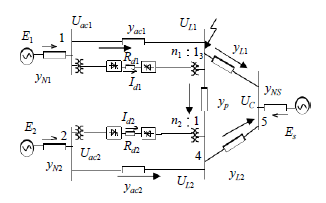Figure 1. Test system model

${A}_{1}{U}_{L1}-{B}_{1}{U}_{L2}={C}_{1}$ (6)

$-{A}_{2}{U}_{L1}+{B}_{2}{U}_{L2}={C}_{2}$ (7)

$\begin{array}{c}{A}_{1}=\left[\left({y}_{L1}+{y}_{L2}+{y}_{NS}\right)\left({y}_{p}+{y}_{N1}\right)+{y}_{L1}\left({y}_{L2}+{y}_{NS}\right)\right]{y}_{ac1}\\ \text{\hspace{0.17em}}\text{\hspace{0.17em}}+{y}_{p}{y}_{N1}\left({y}_{L1}+{y}_{L2}+{y}_{NS}\right)+{y}_{L1}{y}_{N1}\left({y}_{L2}+{y}_{NS}\right)\end{array}$

$\begin{array}{c}{B}_{1}=\left[{y}_{p}\left({y}_{L1}+{y}_{L2}+{y}_{NS}\right)+{y}_{L1}{y}_{L2}\right]{y}_{ac1}\\ \text{\hspace{0.17em}}\text{\hspace{0.17em}}+{y}_{p}{y}_{N1}\left({y}_{L1}+{y}_{L2}+{y}_{NS}\right)+{y}_{L1}{y}_{N1}{y}_{L2}\end{array}$

$\begin{array}{c}{C}_{1}=\left[{y}_{N1}{E}_{1}\left({y}_{L1}+{y}_{L2}+{y}_{NS}\right)+{y}_{L1}{y}_{NS}{E}_{S}\right]{y}_{ac1}\\ \text{\hspace{0.17em}}\text{\hspace{0.17em}}+{y}_{N1}\left({y}_{L1}+{y}_{L2}+{y}_{NS}\right)\frac{\sqrt{6}}{\text{π}}{I}_{d1}+{y}_{L1}{y}_{N1}{y}_{NS}{E}_{S}\end{array}$

$\begin{array}{c}{A}_{2}=\left[{y}_{p}\left({y}_{L1}+{y}_{L2}+{y}_{NS}\right)+{y}_{L1}{y}_{L2}\right]{y}_{ac2}\\ \text{\hspace{0.17em}}\text{\hspace{0.17em}}+{y}_{p}{y}_{N2}\left({y}_{L1}+{y}_{L2}+{y}_{NS}\right)+{y}_{L1}{y}_{N2}{y}_{L2}\end{array}$

$\begin{array}{c}{B}_{2}=\left[\left({y}_{L1}+{y}_{L2}+{y}_{NS}\right)\left({y}_{p}+{y}_{N2}\right)+{y}_{L2}\left({y}_{L1}+{y}_{NS}\right)\right]{y}_{ac2}\\ \text{\hspace{0.17em}}\text{\hspace{0.17em}}+{y}_{p}{y}_{N2}\left({y}_{L1}+{y}_{L2}+{y}_{NS}\right)+{y}_{L2}{y}_{N2}\left({y}_{L1}+{y}_{NS}\right)\end{array}$

$\begin{array}{c}{C}_{2}=\left[{y}_{N2}{E}_{2}\left({y}_{L1}+{y}_{L2}+{y}_{NS}\right)+{y}_{L2}{y}_{NS}{E}_{S}\right]{y}_{ac2}\\ \text{\hspace{0.17em}}\text{\hspace{0.17em}}+{y}_{N2}\left({y}_{L1}+{y}_{L2}+{y}_{NS}\right)\frac{\sqrt{6}}{\text{π}}{I}_{d2}+{y}_{L2}{y}_{N2}{y}_{NS}{E}_{S}\end{array}$

${U}_{L1}=\frac{{C}_{1}{B}_{2}+{B}_{1}{C}_{2}}{{A}_{1}{B}_{2}-{B}_{1}{A}_{2}}$ (8)

${U}_{L2}=\frac{{C}_{1}{A}_{2}+{A}_{1}{C}_{2}}{{A}_{1}{B}_{2}-{B}_{1}{A}_{2}}$ (9)

2.3. 两条直流输电系统传输线导纳与换相电压的关系分析和计算

${A}_{1}={a}_{1}{y}_{ac1}+{a}_{11}$ ${B}_{1}={b}_{1}{y}_{ac1}+{b}_{11}$

${A}_{2}={a}_{2}{y}_{ac2}+{a}_{22}$ ${B}_{2}={b}_{2}{y}_{ac2}+{b}_{22}$

${C}_{1}={c}_{1}{y}_{ac1}+{c}_{11}$ ${C}_{2}={c}_{2}{y}_{ac2}+{c}_{22}$

$\begin{array}{c}{U}_{L1}=\frac{\left({b}_{2}{c}_{1}+{b}_{1}{c}_{2}\right){y}_{ac1}{y}_{ac2}+\left({b}_{22}{c}_{1}+{b}_{1}{c}_{22}\right){y}_{ac1}+\left({b}_{2}{c}_{11}+{b}_{11}{c}_{2}\right){y}_{ac2}+\left({b}_{22}{c}_{11}+{b}_{11}{c}_{22}\right)}{\left({a}_{1}{b}_{2}-{a}_{2}{b}_{1}\right){y}_{ac1}{y}_{ac2}+\left({b}_{22}{a}_{1}-{a}_{22}{b}_{1}\right){y}_{ac1}+\left({a}_{11}{b}_{2}-{a}_{2}{b}_{11}\right){y}_{ac2}+\left({a}_{11}{b}_{22}-{a}_{22}{b}_{11}\right)}\\ =\frac{\left[\left({b}_{2}{c}_{1}+{b}_{1}{c}_{2}\right){y}_{ac2}+\left({b}_{22}{c}_{1}+{b}_{1}{c}_{22}\right)\right]{y}_{ac1}+\left({b}_{2}{c}_{11}+{b}_{11}{c}_{2}\right){y}_{ac2}+\left({b}_{22}{c}_{11}+{b}_{11}{c}_{22}\right)}{\left[\left({a}_{1}{b}_{2}-{a}_{2}{b}_{1}\right){y}_{ac2}+\left({b}_{22}{a}_{1}-{a}_{22}{b}_{1}\right)\right]{y}_{ac1}+\left({a}_{11}{b}_{2}-{a}_{2}{b}_{11}\right){y}_{ac2}+\left({a}_{11}{b}_{22}-{a}_{22}{b}_{11}\right)}\\ =\frac{\left[\left({b}_{2}{c}_{1}+{b}_{1}{c}_{2}\right){y}_{ac1}+\left({b}_{2}{c}_{11}+{b}_{11}{c}_{2}\right)\right]{y}_{ac2}+\left({b}_{22}{c}_{1}+{b}_{1}{c}_{22}\right){y}_{ac1}+\left({b}_{22}{c}_{11}+{b}_{11}{c}_{22}\right)}{\left[\left({a}_{1}{b}_{2}-{a}_{2}{b}_{1}\right){y}_{ac1}+\left({a}_{11}{b}_{2}-{a}_{2}{b}_{11}\right)\right]{y}_{ac2}+\left({b}_{22}{a}_{1}-{a}_{22}{b}_{1}\right){y}_{ac1}+\left({a}_{11}{b}_{22}-{a}_{22}{b}_{11}\right)}\end{array}$ (10)

$\begin{array}{c}{U}_{L2}=\frac{\left({a}_{2}{c}_{1}+{a}_{1}{c}_{2}\right){y}_{ac1}{y}_{ac2}+\left({a}_{22}{c}_{1}+{a}_{1}{c}_{22}\right){y}_{ac1}+\left({a}_{2}{c}_{11}+{a}_{11}{c}_{2}\right){y}_{ac2}+\left({a}_{22}{c}_{11}+{a}_{11}{c}_{22}\right)}{\left({a}_{1}{b}_{2}-{a}_{2}{b}_{1}\right){y}_{ac1}{y}_{ac2}+\left({b}_{22}{a}_{1}-{a}_{22}{b}_{1}\right){y}_{ac1}+\left({a}_{11}{b}_{2}-{a}_{2}{b}_{11}\right){y}_{ac2}+\left({a}_{11}{b}_{22}-{a}_{22}{b}_{11}\right)}\\ =\frac{\left[\left({a}_{2}{c}_{1}+{a}_{1}{c}_{2}\right){y}_{ac2}+\left({a}_{22}{c}_{1}+{a}_{1}{c}_{22}\right)\right]{y}_{ac1}+\left({a}_{2}{c}_{11}+{a}_{11}{c}_{2}\right){y}_{ac2}+\left({a}_{22}{c}_{11}+{a}_{11}{c}_{22}\right)}{\left[\left({a}_{1}{b}_{2}-{a}_{2}{b}_{1}\right){y}_{ac2}+\left({b}_{22}{a}_{1}-{a}_{22}{b}_{1}\right)\right]{y}_{ac1}+\left({a}_{11}{b}_{2}-{a}_{2}{b}_{11}\right){y}_{ac2}+\left({a}_{11}{b}_{22}-{a}_{22}{b}_{11}\right)}\\ =\frac{\left[\left({a}_{2}{c}_{1}+{a}_{1}{c}_{2}\right){y}_{ac1}+\left({a}_{2}{c}_{11}+{a}_{11}{c}_{2}\right)\right]{y}_{ac2}+\left({a}_{22}{c}_{1}+{a}_{1}{c}_{22}\right){y}_{ac1}+\left({b}_{22}{c}_{11}+{b}_{11}{c}_{22}\right)}{\left[\left({a}_{1}{b}_{2}-{a}_{2}{b}_{1}\right){y}_{ac1}+\left({a}_{11}{b}_{2}-{a}_{2}{b}_{11}\right)\right]{y}_{ac2}+\left({b}_{22}{a}_{1}-{a}_{22}{b}_{1}\right){y}_{ac1}+\left({a}_{11}{b}_{22}-{a}_{22}{b}_{11}\right)}\end{array}$ (11)

${U}_{L1}=\frac{{m}_{10}{y}_{ac1}+{h}_{10}}{{m}_{1}{y}_{ac1}+{h}_{1}}$ (12)

${U}_{L2}=\frac{{m}_{20}{y}_{ac1}+{h}_{20}}{{m}_{1}{y}_{ac1}+{h}_{1}}$ (13)

${m}_{10}=\left({b}_{2}{c}_{1}+{b}_{1}{c}_{2}\right){y}_{ac2}+\left({b}_{22}{c}_{1}+{b}_{1}{c}_{22}\right)$

${h}_{10}=\left({b}_{2}{c}_{11}+{b}_{11}{c}_{2}\right){y}_{ac2}+\left({b}_{22}{c}_{11}+{b}_{11}{c}_{22}\right)$

${m}_{1}=\left({a}_{1}{b}_{2}-{a}_{2}{b}_{1}\right){y}_{ac2}+\left({b}_{22}{a}_{1}-{a}_{22}{b}_{1}\right)$

${h}_{1}=\left({a}_{11}{b}_{2}-{a}_{2}{b}_{11}\right){y}_{ac2}+\left({a}_{11}{b}_{22}-{a}_{22}{b}_{11}\right)$

${m}_{20}=\left({a}_{2}{c}_{1}+{a}_{1}{c}_{2}\right){y}_{ac2}+\left({a}_{22}{c}_{1}+{a}_{1}{c}_{22}\right)$

${h}_{20}=\left({a}_{2}{c}_{11}+{a}_{11}{c}_{2}\right){y}_{ac2}+\left({a}_{22}{c}_{11}+{a}_{11}{c}_{22}\right)$

2.4. 两条交流传输线导纳与换相电压的关系分析

$\gamma =\mathrm{arccos}\left[\frac{\sqrt{2}n\cdot {I}_{d}{X}_{L}}{{U}_{L}}+\mathrm{cos}\beta \right]$ (14)

a) 当 ${m}_{1}>0,{h}_{1}>0$ 时，换相电压与传输线导纳的关系如图2所示。

b) 当 ${m}_{1}>0,{h}_{1}<0$ 时，换相电压与传输线导纳的关系如图3所示。

c) 当 ${m}_{1}<0,{h}_{1}>0$ 时，换相电压与传输线导纳的关系如图4所示。

d) 当 ${m}_{1}<0,{h}_{1}<0$ 时，换相电压与传输线导纳的关系如图5所示。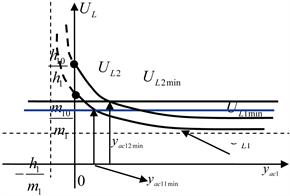Figure 2. Relationship between AC transmission line admittance and commutation voltage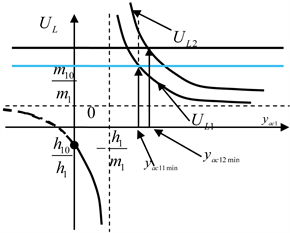Figure 3. Relationship between AC transmission line admittance and commutation voltage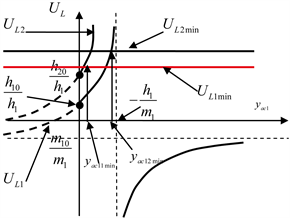Figure 4. Relationship between AC transmission line admittance and commutation voltage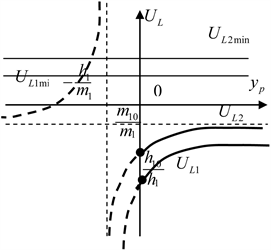Figure 5. Relationship between AC transmission line admittance and commutation voltage

3. 理论分析

$-\frac{{h}_{1}}{{m}_{1}}$ 后，此时两直流输电子系统的分析同图2所示的分析一样有相同的规律。

4. 模型的故障仿真和分析

4.1. 模型系统的初始运行值

${U}_{L10}=525\text{\hspace{0.17em}}\text{kV},{\beta }_{10}={132}^{\circ },{n}_{10}=1.0\left(\text{p}\text{.u}\text{.}\right),{I}_{d10}=1.0\left(\text{p}\text{.u}\text{.}\right)=2\text{\hspace{0.17em}}\text{kA},{L}_{cr10}=52.2\text{\hspace{0.17em}}\text{mH}$

${U}_{L20}=525\text{\hspace{0.17em}}\text{kV},{\beta }_{20}={142}^{\circ },{n}_{20}=1.0\left(\text{p}\text{.u}\text{.}\right),{I}_{d20}=1.0\left(\text{p}\text{.u}\text{.}\right)=2\text{\hspace{0.17em}}\text{kA},{L}_{cr20}=56.2\text{\hspace{0.17em}}\text{mH}$

$\begin{array}{l}{y}_{ac1}=0.05818\text{\hspace{0.17em}}{\Omega }^{-1},\text{\hspace{0.17em}}{y}_{ac2}=0.01358\text{\hspace{0.17em}}{\Omega }^{-1}\\ {y}_{L1}=0.01758\text{\hspace{0.17em}}{\Omega }^{-1},\text{\hspace{0.17em}}{y}_{L2}=0.02759\text{\hspace{0.17em}}{\Omega }^{-1}\\ {y}_{p}=0.01758\text{\hspace{0.17em}}{\Omega }^{-1}\end{array}$

${E}_{1}=1.34638\left(\text{p}\text{.u}\text{.}\right),{E}_{2}=1.3522\left(\text{p}\text{.u}\text{.}\right)$

$\begin{array}{l}{y}_{N1}=3.1152{\Omega }^{-1}\\ {y}_{N2}=3.1152{\Omega }^{-1}\end{array}$

${y}_{Ns}=2.94118{\Omega }^{－1}$

${E}_{s}=1.5235\left(\text{p}\text{.u}\text{.}\right)$

${U}_{L1}=\frac{141.94{y}_{ac1}+2.8286}{-0.32763{y}_{ac1}+0.143216}$ (15)

${U}_{L2}=\frac{141.94{y}_{ac1}+3.2027}{-0.32763{y}_{ac1}+0.143216}$ (16)

${U}_{L1\mathrm{min}}=105\left(\text{kV}\right),{U}_{L2\mathrm{min}}=97.85\left( kV \right)$

$\begin{array}{l}{y}_{ac11\mathrm{min}}=0.06923\text{\hspace{0.17em}}{\Omega }^{-1}\\ {y}_{ac12\mathrm{min}}=0.06428\text{\hspace{0.17em}}{\Omega }^{-1}\end{array}$

4.2. 逆变侧交流母线故障仿真和分析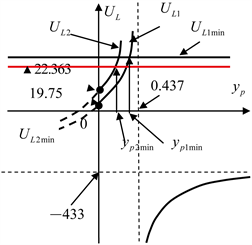Figure 6. Relationship between AC transmission line admittance and commutation voltage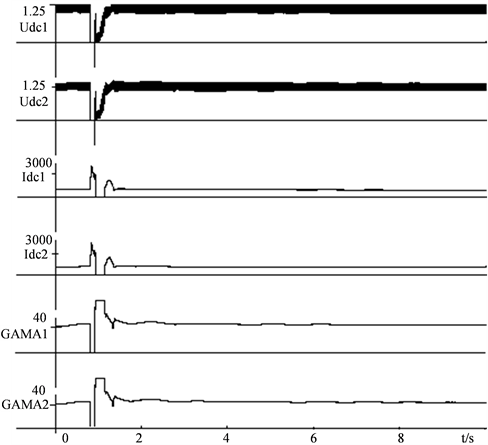Figure 7. Systems simulation responses of Uac1 Uac2, Idc1, Idc2, Gama1, Gama2

5. 结论

1) 本文从理论上分析了与多馈入直流输电系统并联的传输线导纳和影响直流系统是否会同时或相继发生换相失败的关系；

2) 值得指出的是，影响多馈入直流输电系统中换相失败发生的主要因素是由网络结构决定的。它是与各直流输电子系统相并联的交流传输线的导纳以及与各直流输电子系统整流侧相连的交流电源的等值导纳和与各直流输电子系统逆变侧相连的交流电源的等值导纳密切相关；

3) 本文的仿真模型是一个典型的多馈入交、直流并联的现代电力系统的基本结构模型。模型仿真也验证了上面理论分析所得结论判断换相失败的准确性和正确性。对于实际的大规模交、直流并联的电力系统均可以通过适当的等值处理为该模型。因此该模型具有一定的科研和教学意义。

 荆勇, 李立涅, 任震. 天广交直流系统并联运行的稳定控制[J]. 电力系统自动化, 2002, 26(1): 49-52.

 欧开健, 任震, 荆勇等. 直流输电系统换相失败的研究(一) [J]. 电力自动设备, 2003, 23(5).

 任震, 欧开健, 荆勇等. 直流输电系统换相失败的研究(二) [J]. 电力自动设备, 2003, 23(6).

 赵畹君. 高压直流输电工程技术[M]. 北京: 中国电力出版社, 2004: 127-131.

 项玲. 郑建勇, 等. 多端和多馈入直流输电系统中换相失败的研究[J]. 电力系统自动化, 2005, 29(11): 29-33.

 毛晓明, 管霖, 张尧, 吴小辰, 彭显刚. 含有多馈入直流的交直流混合电网高压直流建模研究[J]. 中国电机工程学报, 2004, 24(9): 68-73.

 陈树勇, 李新年, 余军, 李涛, 吕鹏飞, 印永华. 基于正余弦分量检测的高压直流换相失败预防方法[J]. 中国电机工程学报, 2005, 25(14): 1-6.

 Hansen, A. and Havemann, H. (2000) Decreasing the Commutation Failure Frequency in HVDC Transmission Systems. IEEE Transactions on Power Delivery, 15, 1022-1026.
https://doi.org/10.1109/61.871369

 李兴源. 高压直流输电系统的运行和控制[M]. 北京: 科学出版社, 1998.

 PRABHA KUNDUR. 电力系统稳定和控制[M]. 北京: 中国电力出版社, 2002.

 杨卫东, 徐政, 韩祯祥. NETOMAC在直流输电系统仿真研究中的应用[J]. 电力自动化设备, 2001, 21(4): 10-14.

 Zhou, C.C. and Xu, Z. (2002) Study on Commutation Failure of Multi-Infeed HVDC system. Proceedings of International Conference on Power System Technology, 4, 2462-2466.
https://doi.org/10.1109/ICPST.2002.1047228

 Taylor, C.W. and Hauer, J.F. (1989) Supplementary Controls in a Power System with Parallel AC and DC Transmission. CIGRE International Colloquium on HVDC Power Transmission. Recife, Brasil. 13-15 August 1989, I-06.

 何朝荣, 李兴源, 金小明, 等. 高压直流输电系统换相失败判断标准的理论分析[J]. 电网技术, 2006, 30(22).

 吴红斌, 丁明, 刘波. 交直流系统暂态仿真中换流器的换相过程分析[J]. 电网技术, 2004, 28(17): 11-14.

 Thio, C.V., Davies, J.B. and Kent, K.L. (1996) Commutation Failures in HVDC Transmission Systems. IEEE Transactions on Power Delivery, 11, 946-957.

Top